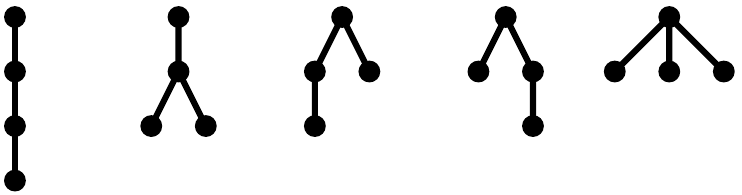###### back to index | new

Let $n$ be a positive integer and $N=\displaystyle\sum_{k=0}^{n}(-1)^k\binom{n}{k}^2$. Show that $N=0$ if $n$ is odd, and $N=(-1)^{\frac{n}{2}}\displaystyle\binom{n}{\frac{n}{2}}$ if $n$ is even.

Show that $$\sum_{k=0}^{n}(-1)^k\frac{m}{m+k}\binom{n}{k}=\frac{1}{\binom{m+n}{n}}$$

Let the binary representation of positive integer $n$ be $b_tb_{t-1}\cdots b_1b_0$. Show that $$\binom{n}{2^j} \equiv b_j \pmod{2}$$

where $j$ is a non-negative integer. Note that $\binom{n}{m} = 0$ if $m > n$.

Let $n$ be a positive integer and $k$ be the number of $1$s in $n$'s binary representation. Show there are $2^k$ odd integers in $\binom{n}{0}$, $\binom{n}{1}$, $\cdots$, $\binom{n}{n}$.

Calculate the value of $$\sum_{k=1}^{2n-1}(-1)^{k-1}\binom{2n}{k}^{-1}$$

Show that $$\left(\sum_{k=0}^{\infty}x^k\right)^2=\sum_{k=0}^{\infty}(k+1)x^k$$

Let $f(x)$ be the generating function for $a_0$, $a_1$, $a_2$, $\cdots$. Find the generating function for $$a_0, a_0 + a_1, a_0+a_1+a_2, \cdots$$

Let $n$ be a positive integer greater than $1$. Show $$\sum_{k=1}^{n-1}\frac{1}{k(n-k)}\binom{2(k-1)}{k-1}\binom{2(n-k-1)}{n-k-1}=\frac{1}{n}\binom{2(n-1)}{n-1}$$

Show $$\sum_{k=0}^{n}(-1)^k\binom{n}{k}\binom{m+k}{q}=(-1)^n\binom{m}{q-n}$$

Given randomly selected $5$ distinct positive integers not exceeding $90$, what is the expected average value of the fourth largest number?

For every integer $n$ from $0$ to $6$, we have $3$ identical weights with weight $2^n$ grams. How many total ways are there to form a total weight of $263$ grams using only these weights?

How many different ways are there to make a payment of $n$ dollars using any number of $\$1$and$\$2$ bills?

There are $10$ red, $10$ blue, and $10$ white balls. How many different ways are there to retrieve $16$ balls with all the three colors present.

Let $\alpha(n)$ be the number of ways to write a positive integer $n$ as the sum of $1$s and $2$s. Let $\beta(n)$ be the number of ways to write $n$ as a sum of several integers greater than $1$. Different orders are treated as different. Prove $\alpha(n)=\beta(n+2)$.

Compute the value of $$\sum_{k=0}^{n}\frac{1}{2^k}\binom{n+k}{n}$$

Show that $$\frac{1}{1-x^2}=\sum_{k=0}^{\infty}x^{2k}$$

Show that $$\frac{1}{(1-ax)^n}=\sum_{k=0}^{\infty}a^k\binom{n-k}{n-1}x^k$$

Seven students took a math exam. Every problem was solved at at most $3$ students. For every pair of students, there is at least one problem which was solved by both of these two students. What is the minimal number of problem this exam contains?

Let $n$ be a positive integer. Find the number of paths from $(0,0)$ to $(n,n)$ on a $n\times n$ grid using only unit up and right steps so that the path stays in the region $x\ge y$.

Find the number of expressions containing $n$ pairs of parentheses which are correctly matched. For example, when $n=3$, the answer is $5$ because all the valid expressions are $$((())),\ (()()),\ (())(),\ ()(()),\ ()()()$$

A tree is a structure which grows from a single root node. By convention, the root node is usually placed at the top. Then, new nodes, referred as children nodes, can be attached to an existing node, referred as parent node, by edges. The root node has no parent and all other nodes have exactly one parent. A node may have any number of children nodes, including no child at all. No looping chain of nodes is permitted in this structure. For example, there are exactly $5$ different types of trees with $4$ nodes. They are shown below. The goal is to find the number of different types of tree with $n$ nodes where $n$ is a positive integer greater than $1$.Find the generating function for the sequence $\ 0,\ 1,\ -\frac{1}{2},\ \frac{1}{3},\ -\frac{1}{4},\ \cdots$

There are $30$ identical souvenirs to be distributed among $50$ students. Each student may receive more than one souvenir. How many different distribution plans are there?

Find the number of integer solutions to the equation $a+b+c=6$ where $-1 \le a < 2$ and $1\le b,\ c\le 4$.

Let positive integers $n$ and $k$ satisfy $n\ge 2k$. How many $k$-sided convex polygons are there whose vertices are those of an $n$-sided convex polygon and edges are diagonals of the same $n$-polygon.